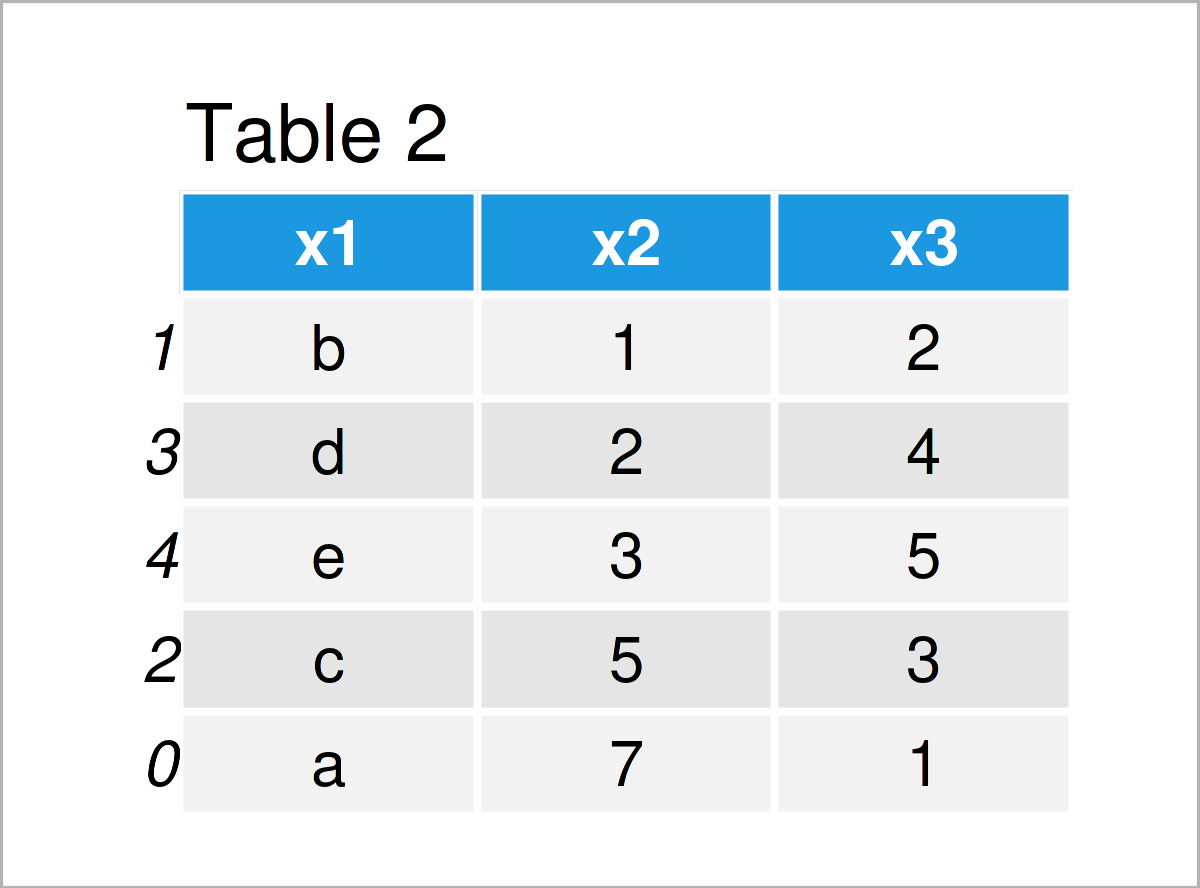# Sort pandas DataFrame by Column in Python (Example)

This tutorial explains how to order a pandas DataFrame by the values in a column in the Python programming language.

The tutorial contains this information:

Sound good? Let’s dig in:

## Example Data & Software Libraries

In order to use the functions of the pandas library, we first need to load pandas:

`import pandas as pd # Load pandas library`

As a next step, let’s also construct some example data:

```data = pd.DataFrame({'x1':['a', 'b', 'c', 'd', 'e'], # Create example DataFrame 'x2':[7, 1, 5, 2, 3], 'x3':range(1, 6)}) print(data) # Print example DataFrame```Have a look at the previous table. It visualizes that our exemplifying data contains five rows and the three columns “x1”, “x2”, and “x3”.

## Example: Ordering Rows of pandas DataFrame Based On Values in Column

In this example, I’ll demonstrate how to sort the rows of a pandas DataFrame according to the values that are stored in one of the columns of this DataFrame.

For this task, we can use the sort_values function as shown below:

```data_new = data.copy() # Duplicate DataFrame data_new = data_new.sort_values('x2') # Order DataFrame print(data_new) # Print updated DataFrame```As shown in Table 2, we have created a new pandas DataFrame called data_new by running the previous Python code.

As you can see, the rows of this DataFrame are rearranged by the variable x2.

## Video & Further Resources

Have a look at the following video on the DataDaft YouTube channel. In the video instruction, the speaker explains in another example how to order the rows of a pandas DataFrame by a column in Python.

Please accept YouTube cookies to play this video. By accepting you will be accessing content from YouTube, a service provided by an external third party.If you accept this notice, your choice will be saved and the page will refresh.

Furthermore, you might read the other articles on my homepage. You can find some other tutorials below.

Summary: This post has shown how to sort the rows of a pandas DataFrame by the values in a column in the Python programming language. Please let me know in the comments, if you have further questions. Furthermore, don’t forget to subscribe to my email newsletter to get updates on the newest tutorials.

Subscribe to the Statistics Globe Newsletter